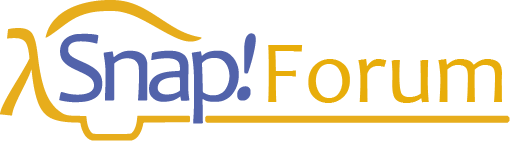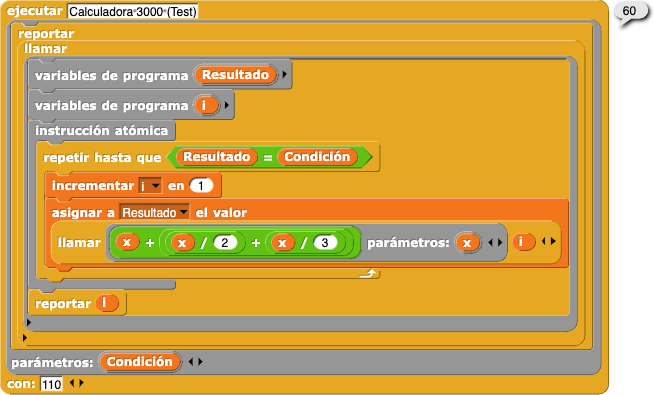# Today I will show you something new for ideas for Snap!

Since my native language is spanish, the blocks had to be adapted to my language. Also my idea was thanks to an mathematical problem, but at that moment I had to create a solution for that.x + x/2 + x/3 = 110
this is the real result:
x = 60
60 + 60/2 + 60/3 =110 or 60 + 30 + 20 = 110
Then x is the parameter to calculate the result.
The result is the condition.

Snap! Blocks Style:

snap!_blocks
(call [
script variables (i)
warp
start
start
change [i] in (1)
set [Resultado] to (call [((x) + (((x) / (2)) + ((x) / (3))))] with parameters: (x) < > ] (i) < > )
end
end
return (i) > ]
>
with parameters: (Condición) < > ]
with: (110) < > )
snap!_blocks

Could I suggest that you stop using Snap 7.0 in your postsWhen the real Snap! 7.0 comes out - google might get confused with the real version and your suggestionsI suggest saying "future Snap version" instead